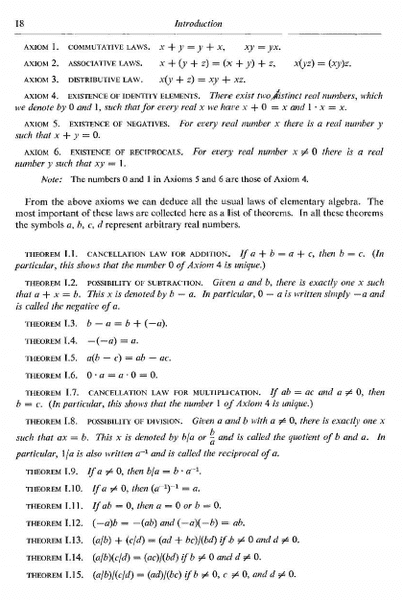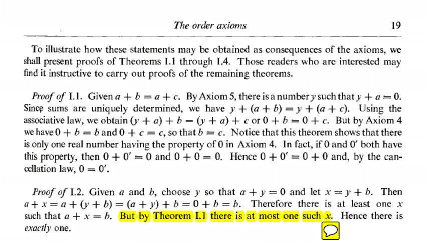# Need help understanding text from 'Calculus' by Apostol

• I
• omoplata

#### omoplata

Sorry if I'm posting in the wrong place. Since the difficulty I have is with the text of the book, and not the exercises, I posted here.

In proving theorem I.2, how is theorem I.1 used to assert that 'there is at most one such x'? The first image below gives the background text. The text I have trouble with is highlighted in the second image below.Thank you.

Assume that x and y satisfy theorem 1.2.

That means that:
a + x = b
a + y = b

What can you deduce from that about a + x and a + y?

•Astyute Chick
Assume that x and y satisfy theorem 1.2.

But I can't assume that. Theorem I.2 is the one I'm trying to prove.

But I can't assume that. Theorem I.2 is the one I'm trying to prove.
You are questioning the part of theorem 1.2 that 'there is at most one such x'.
You prove that by assuming that there are two, and then (hopefully), conclude that they must be equal.
That in itself indeed doesn't prove that "there exists" an x satisfying theorem 1.2, but that if one exists, it is unique.
Do you see how that uniqueness follows from theorem 1.1?

•Astyute Chick
Oh, I see now.

So I have come up to the point saying a + x = b.

But I do not know yet if x is unique. So I assume there is a another y such that a + y = b.

Then, a + x = a + y

From theorem I.1, x = y.

Therefore x is unique.

Thank you!

•Astyute Chick and Samy_A
Assume that x and y satisfy theorem 1.2.

That means that:
a + x = b
a + y = b

What can you deduce from that about a + x and a + y?

Maybe this will help for future reference. In mathematics, when we are trying to show uniqueness. We usually do this by assuming that there are two things that have a given property. Then we find a contradiction.

If you have taken linear algebra. A good example would be the uniqueness of the additive identity of a vector space, the 0 vector.

Your post #5, is a great idea to think about when we want to show uniqueness.

One more thing. If you are able to show existence and uniqueness, we get an iff statement.

Maybe this will help for future reference. In mathematics, when we are trying to show uniqueness. We usually do this by assuming that there are two things that have a given property. Then we find a contradiction.

If you have taken linear algebra. A good example would be the uniqueness of the additive identity of a vector space, the 0 vector.

Your post #5, is a great idea to think about when we want to show uniqueness.

Rather assuming that if two things satisfy a given property, then they are equal. Sometimes contradiction can be used, but most of the times it isn't necessary (in your example of the additive identity it isn't necessary: if 0,0' are identities, it follows that 0 + 0' = 0 = 0' + 0 and 0+0' = 0' = 0' + 0, in particular thus 0=0')

Rather assuming that if two things satisfy a given property, then they are equal. Sometimes contradiction can be used, but most of the times it isn't necessary (in your example of the additive identity it isn't necessary: if 0,0' are identities, it follows that 0 + 0' = 0 = 0' + 0 and 0+0' = 0' = 0' + 0, in particular thus 0=0')
That is why i said usually. However, you were correct with the "rather assuming ..." part. I chose the example of the additive identity, because it shows how to start a uniqueness proof. The contradiction part was added, because there are a few theorems and exercises coming up in Apostol that the OP will need to know this idea.

•member 587159